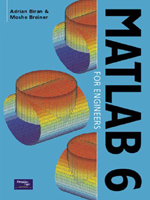MATLAB 6 for Engineers:
An Introduction

By Adrian Biran & Moshe Breiner
August 2002
Prentice Hall / Pearson Education
ISBN: 0-13-033631-9797 Pages, Illustrated
\$115.00 paper original

MATLAB 6 is the latest version of the popular, comprehensive and easy-to-use environment for performing technical and scientific calculations. Its interactive nature offers invaluable assistance with numeric computation and data visualization. MATLAB 6 for Engineers provides a clear and concise introduction to MATLAB 6, demonstrating its enhanced programming capabilities and exploring its use in solving engineering problems.

Written for engineering students and practicing engineers, it shows how MATLAB can be used to perform calculations, store tables and interpolate between stored values, process numbers obtained by data acquisition systems, and prepare engineering and research reports. The book is split into three parts- Chapter 1 is a concise MATLAB tutorial for users wishing to get quickly acquainted with the package itself; Part I (Chapters 2-10) is an introduction for engineers, beginning with basic calculations and helping the user to master techniques for solving progressively more complex problems; Part II (Chapters 11-18) focuses on more advanced material, featuring specialized applications and new functions.

Key features include: Full coverage of the main features of MATLAB 6. A guided introduction to the basic MATLAB functions . Written in a clear and readable style. A wealth of end of chapter exercises, with solutions to selected problems. Real world examples from engineering mathematics, mechanical and electrical engineering. Appendices and summaries to reinforce learning. Studies of classical engineering problems, for which MATLAB yields concise and elegant solutions.

Adrian Biran is an Adjunct Associate Professor in the Faculty of Mechanical Engineering at Technion, Israel Institute of Technology.. Moshe Breiner is the Managing Director of SimACon, a company specializing in the fields of simulation, mathematical analysis and controls.